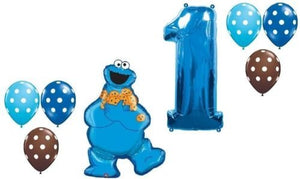# Loonballoon Cookie Monster Sesame Polka Dots #1 1St Birthday Party Mylar Latex Balloon Set

Vendor
LoonBalloon
Regular price
\$42.97
Sale price
\$42.85
Quantity must be 1 or more

• L

• o

• o

• n

• B

• a

• l

• l

• o

• o

• n

• C

• O

• O

• K

• I

• E

• M

• O

• N

• S

• T

• E

• R

• S

• e

• s

• a

• m

• e

• P

• o

• l

• k

• a

• D

• o

• t

• s

• #

• 1

• 1

• s

• t

• B

• i

• r

• t

• h

• d

• a

• y

• P

• A

• R

• T

• Y

• M

• y

• l

• a

• r

• L

• a

• t

• e

• x

• B

• A

• L

• L

• O

• O

• N

• S

• e

• t

LoonBalloon COOKIE MONSTER Sesame Polka Dots #1 1st Birthday PARTY Mylar Latex BALLOON SetGreat for parties, birthdays, holidays, and special occasions. Mix and match with latex and foil balloons to create fun, festive balloon bouquets for easy party decorations and fun!